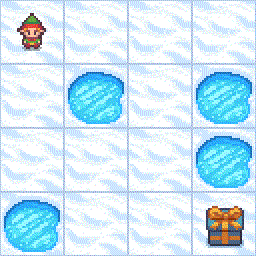#!/usr/bin/env python # coding: utf-8 # # Q* Learning - FrozenLake #
# In this Notebook, we'll implement an agent that plays FrozenLake. ## # The goal of this game is to go from the starting state (S) to the goal state (G) by walking only on frozen tiles (F) and avoid holes (H).However, the ice is slippery, so you won't always move in the direction you intend (stochastic environment) # # # Reference: code adapted from # https://github.com/simoninithomas/Deep_reinforcement_learning_Course/blob/master/Q%20learning/FrozenLake/Q%20Learning%20with%20FrozenLake.ipynb # In: #!pip install gym #!pip install pygame #!pip install gym[toy_text] # In: import numpy as np import gymnasium as gym #to load the FrozenLake Environment import random import matplotlib.pyplot as plt from tqdm import tqdm # In: #env = gym.make("FrozenLake-v0") env = gym.make("FrozenLake-v1",is_slippery=True, render_mode="rgb_array")#creates the FrozenLake environment using the gym library of environments # In: print(env.reward_range, env.metadata, env.spec, env.action_space.contains(2)) #env.desc # ## Step 2: Create the Q-table and initialize it # In: action_size = env.action_space.n#how many actions there are state_size = env.observation_space.n#how many states there are qtable = np.zeros((state_size, action_size)) print(qtable) # ## Set the hyperparameters # In: total_episodes = 100000 # Total episodes learning_rate = 0.1 # Learning rate max_steps = 99 # Max steps per episode gamma_rate = 0.95 # Discounting rate # Exploration parameters epsilon = 0.1 # Exploration rate max_epsilon = 0.5 # Exploration probability at start min_epsilon = 0.01 # Minimum exploration probability decay_rate = 0.00 # Exponential decay rate for exploration prob # ## Step 4: The Q learning algorithm # - Now we implement the Q learning algorithm: ## In: # List of rewards rewards = [] for episode in tqdm(range(total_episodes)): # Reset the environment state,_= env.reset() step = 0 done = False total_rewards = 0 for step in range(max_steps): # epsilon-greedy strategy exploration_or_exploitation = random.uniform(0, 1) ## If this number > greater than epsilon --> exploitation (taking the biggest Q value for this state) if exploration_or_exploitation > epsilon: action = np.argmax(qtable[state,:]) # Else doing a random choice --> exploration else: action = env.action_space.sample() # Take the action (a) and observe the outcome state(s') and reward (r) new_state, reward, terminated, truncated, info = env.step(action) # Update Q(s,a):= Q(s,a) + lr [R(s,a) + gamma * max Q(s',a') - Q(s,a)] # qtable[new_state,:] : all the actions we can take from new state qtable[state, action] += learning_rate * (reward + gamma_rate * np.max(qtable[new_state, :]) - qtable[state, action]) total_rewards += reward # Our new state is state state = new_state # If done (if we're dead) : finish episode if terminated|truncated: break # Schedule to reduce epsilon epsilon = min_epsilon + (max_epsilon - min_epsilon)*np.exp(-decay_rate*episode) rewards.append(total_rewards) print ("Score over time: " + str(sum(rewards)/total_episodes)) print(qtable) # In: # visualisation de la table Q pour FrozenLake def plotQ(q_table,env): MAP=env.desc map_size=MAP.shape best_value = np.max(q_table, axis = 1).reshape((map_size,map_size)) best_policy = np.argmax(q_table, axis = 1).reshape((map_size,map_size)) fig, ax = plt.subplots() im = ax.imshow(best_value,cmap=plt.cm.Wistia)#Pastel1, spring, autumn arrow_list=['<','v','>','^'] for i in range(best_value.shape): for j in range(best_value.shape): if MAP[i][j].decode('utf-8') in 'GH':#terminal states arrow = MAP[i][j].decode('utf-8') else : arrow=arrow_list[best_policy[i, j]] if MAP[i][j].decode('utf-8') in 'S': arrow = 'S ' + arrow text = ax.text(j, i, arrow, ha = "center", va = "center", color = "black") text2 = ax.text(j, i+0.2, str(np.round(best_value[i,j],2)), ha = "center", va = "center",color = "blue") cbar = ax.figure.colorbar(im, ax = ax) plt.axis('off') fig.tight_layout() plt.show() plotQ(qtable,env) # In: #print(qtable) #np.max(qtable, axis = 1).reshape((4,4)) #look at https://gsverhoeven.github.io/post/frozenlake-qlearning-convergence/ for the optimal value function # ## Step 5: Use the Q-table to play FrozenLake # - After 10 000 episodes, our Q-table can be used as a "cheatsheet" to play FrozenLake" # - By running this cell you can see our agent playing FrozenLake. # In: rewards = [] total_test_episodes=10 for episode in range(total_test_episodes): state,_ = env.reset() step = 0 done = False total_rewards = 0 print("****************************************************") print("EPISODE ", episode) for step in range(max_steps): # Take the action (index) that have the maximum expected future reward given that state action = np.argmax(qtable[state,:]) #env.render() new_state, reward, term,trunc, info = env.step(action) total_rewards +=reward if term|trunc: # Here, we decide to only print the last state (to see if our agent is on the goal or fall into an hole) plt.imshow(env.render()) # We print the number of step it took. print("Number of steps", step) break state = new_state rewards.append(total_rewards) print ("Score over time: " + str(sum(rewards)/total_test_episodes)) env.close() # ##Questions # # - is the obtained value function optimal ? # - is the obtained policy optimal ? # - when $\gamma$ goes to $1.0$ do the policy changes ? # - change the total number of training episodes # - implement Double Q-learning ... # # In[ ]: